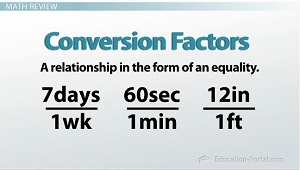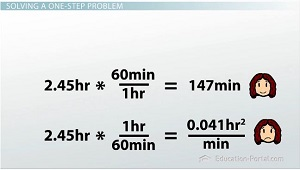# Unit Conversion and Dimensional Analysis

An error occurred trying to load this video.

Try refreshing the page, or contact customer support.

Coming up next: Significant Figures and Scientific Notation

### You're on a roll. Keep up the good work!

Replay
Your next lesson will play in 10 seconds
• 0:06 Dimensional Analysis
• 1:31 Math Review
• 3:39 Solving a One-Step Problem
• 5:03 Solving a Multi-Step Problem
• 8:21 Canceling Numerator &…
• 9:45 Lesson Summary
Save Save

Want to watch this again later?

Timeline
Autoplay
Autoplay
Speed Speed

#### Recommended Lessons and Courses for You

Lesson Transcript
Instructor: Kristin Born

Kristin has an M.S. in Chemistry and has taught many at many levels, including introductory and AP Chemistry.

How is solving a chemistry problem like playing dominoes? Watch this lesson to find out how you can use your domino skills to solve almost any chemistry problem.

## Dimensional Analysis

How many minutes are in 2.45 hours? I know this doesn't seem like a chemistry question, but in this lesson, we're going to learn a technique that is used to solve nearly every type of chemistry problem. This technique has several different names, but the most common are dimensional analysis and the factor-label method. Dimensional analysis is a simple tool for solving problems not just in chemistry, but in everyday life. It allows us to convert a number from one unit to another unit. If you ever need to figure out how much carpet to buy for your living room, how much it will cost, or how long it will take to travel somewhere, you can use dimensional analysis. Not only will using it help you stay organized as you solve problems, but it will likely reduce the risk of errors by providing a way to double-check your work.

So let's go back to our initial question: how many minutes are in 2.45 hours? My number-one piece of advice for answering a question like this is to try not to do it in your head or take any shortcuts. It may seem like an easy question to answer by punching numbers into a calculator, but when the problems start getting more complicated and more abstract, you will be more likely to make mistakes if you don't use this method to solve problems.

## Math ReviewLet's start the process of answering this question by first reviewing three basic math rules:

1. First of all, what do you get when you multiply a number, let's just say x, by 1? Hopefully, you said 'the original number' or x. Multiplying by 1 does not change the value of the original number. You can always multiply by 1 without changing a number's value.

2. Next, let's take a look at some of the properties of fractions. You would solve 2/4 * 5/2 by multiplying across the top (to get 10) and across the bottom (to get 8), but because you have the same value (2) in both the numerator and the denominator, these numbers can cancel out, reducing the amount of work you would need to do in the end by simplifying the 10/8 fraction to 5/4. But what if you have something like 5 cm * 1 m/100 cm? Can you cancel out the units as well? Yes! Identical numbers and units on the top and bottom of multiplied fractions will cancel each other out.

3. Finally, we need to examine what a measurement really is. If a reaction takes 1 hour, there are several different ways we can record this: 1 hour, 60 minutes, 3,600 seconds, and so on. There are multiple ways to write a measurement without changing its value. In dimensional analysis, we will use conversion factors to express these equalities. A conversion factor is a relationship in the form of an equality. For example, 7 days/1 week, 60 seconds/1 minute, or 12 inches/1 foot are all examples of conversion factors. Some conversion factors may be difficult to identify. For example, the density of aluminum is 2.7 g/cm3.

## Solving a One-Step Problem

How do all these rules help us solve our problem converting 2.45 hours into minutes? We will first want to start out with the quantity we are given. Then, we will need to set up a conversion factor (or a group of conversion factors) that will allow us to solve the problem. When setting up a conversion factor for this problem, we need to identify our known equalities. In this situation, we know that 1 hour is equivalent to 60 minutes. We can either write our conversion factor as 60 minutes/1 hour or 1 hour/60 minutes. We are going to need to choose one of these conversion factors to help answer our question, so which one do we use?

Let's see what happens with each one. If we take 2.45 hours and multiply by 1 hour/60 minutes (which is equal to 1), we get a value of 0.041 hours2 /minutes. If we take 2.45 hours and multiply by 60 minutes/1 hour (also equal to 1), we get a value of 147 minutes. If we compare these two answers, we need to understand that both of them are equivalent values, but one gives you the units you want, and one does not.## Solving a Multi-Step Problem

Let's take a slightly more challenging example: convert 2.3 miles into centimeters. First, let's list our conversion factors (the information we will most likely be using to solve this problem). We have 1 mile = 5,280 feet, 1 foot = 12 inches, and 1 inch = 2.54 centimeters. There may be some conversion factors you will need to memorize, but we will discuss those as needed throughout the course. For now, just focus on setting up the problem using the conversion factors. This is where it gets fun.

We can solve this almost like we would play a game of dominoes. If you are given the tile shown (3/6) and you have tiles a (0/5), b (6/0), c (2/5), and d (4/5) in your possession, you will need to place them in an order that allows you to connect to the 2/3 on the right side of the sequence. This may take a little trial-and-error and a little problem-solving, but you should come up with this sequence: tile b, tile a, tile c. You may think you could use either tile c or tile d for the last-placed tile, but tile d wouldn't work because it doesn't connect up with the 2 on the last tile.

To unlock this lesson you must be a Study.com Member.

### Register to view this lesson

Are you a student or a teacher?

#### See for yourself why 30 million people use Study.com

##### Become a Study.com member and start learning now.
Back
What teachers are saying about Study.com

### Earning College Credit

Did you know… We have over 200 college courses that prepare you to earn credit by exam that is accepted by over 1,500 colleges and universities. You can test out of the first two years of college and save thousands off your degree. Anyone can earn credit-by-exam regardless of age or education level.